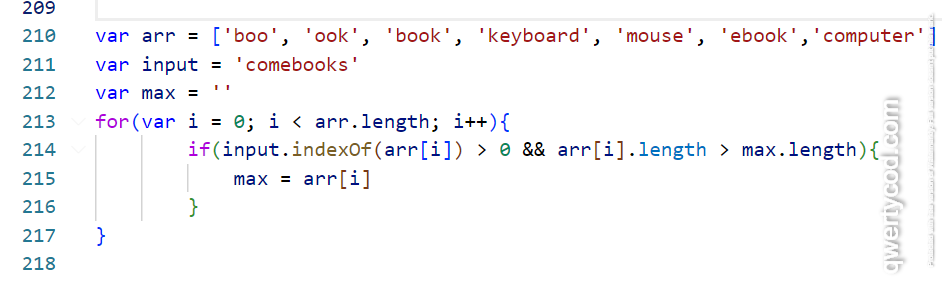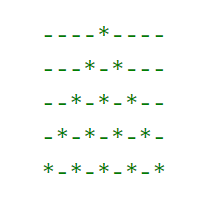# JavaScript coding interview questions (2023), experience 2-5 years

## Programming questions

Questions are targeted for 2-5 years of experience but can be tried by beginners also, they may take more time.

Average time to complete the questions ranges from 5 minutes to 12 minutes max.1 => Write a program to check if there is a comma (,) at the end of a string, and if its present at the end then remove this last comma (,) only from the string, not all the commas. (Program is to be written without using the inbuild Trim() method )

s = “aabbcc,dd,ee,”
Output should be:  “aabbcc,dd,ee”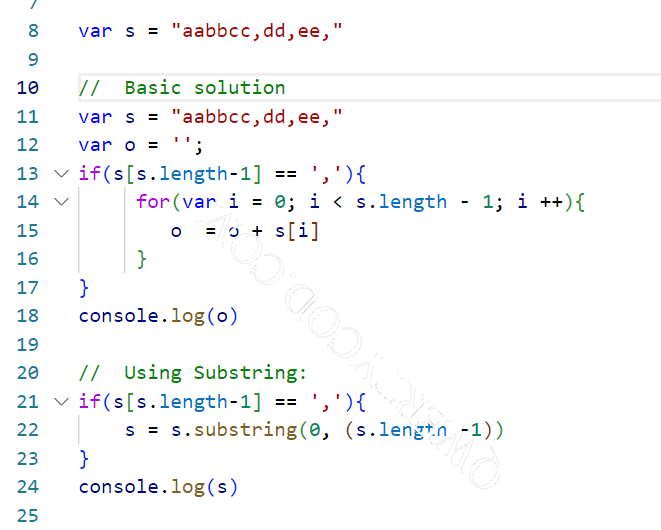2 => Find last n elements from the given array

function getLastEelementOfArray( n, arr) {
}

For input – getLastEelementOfArray(3, [4, 2, 9, –1])

Output should be [2,9,-1], it contains the last 3 elements from the array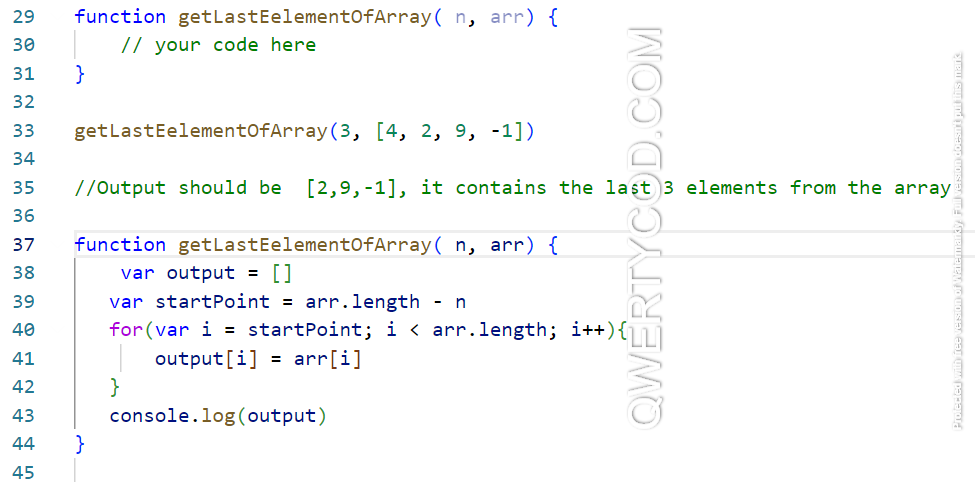3 => Get distinct values in the array
var input = [‘a’, ‘b’, ‘c’, ‘d’, ‘a’,’d’, ‘a’,’b’, ‘c’,’e’,’a’]
// output should be [‘a’, ‘b’, ‘c’, ‘d’, ‘e’]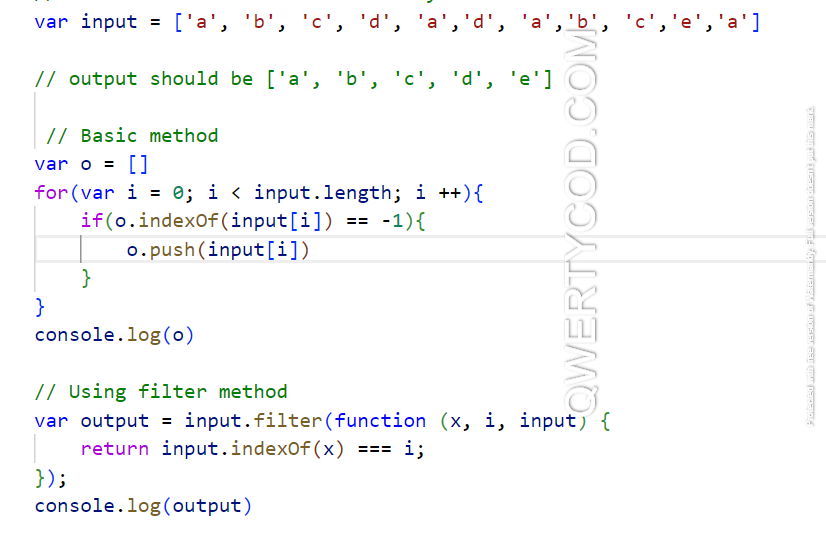4 => Conceptual question, Hoisting, (var vs let)
What will be output of below code: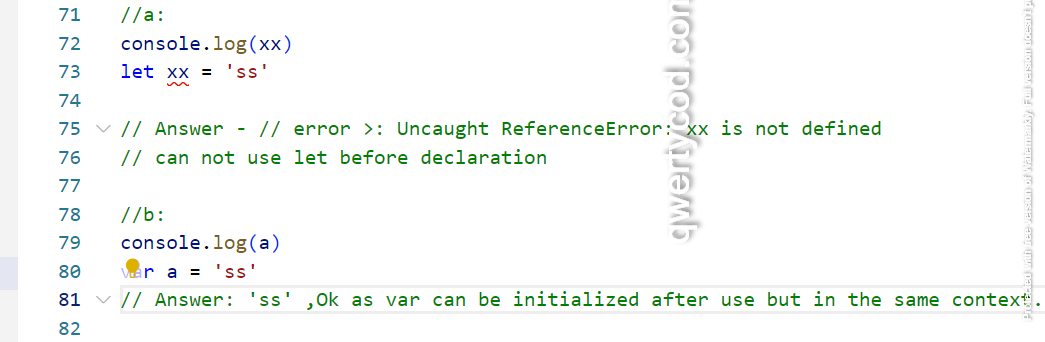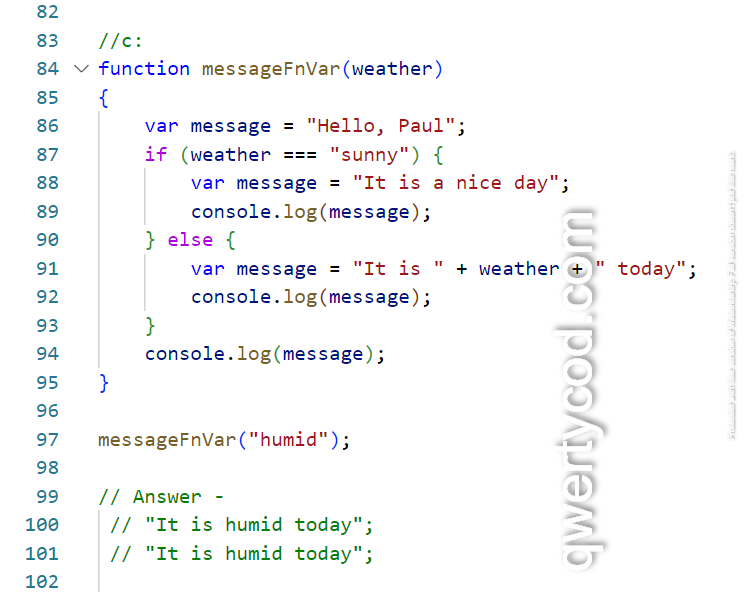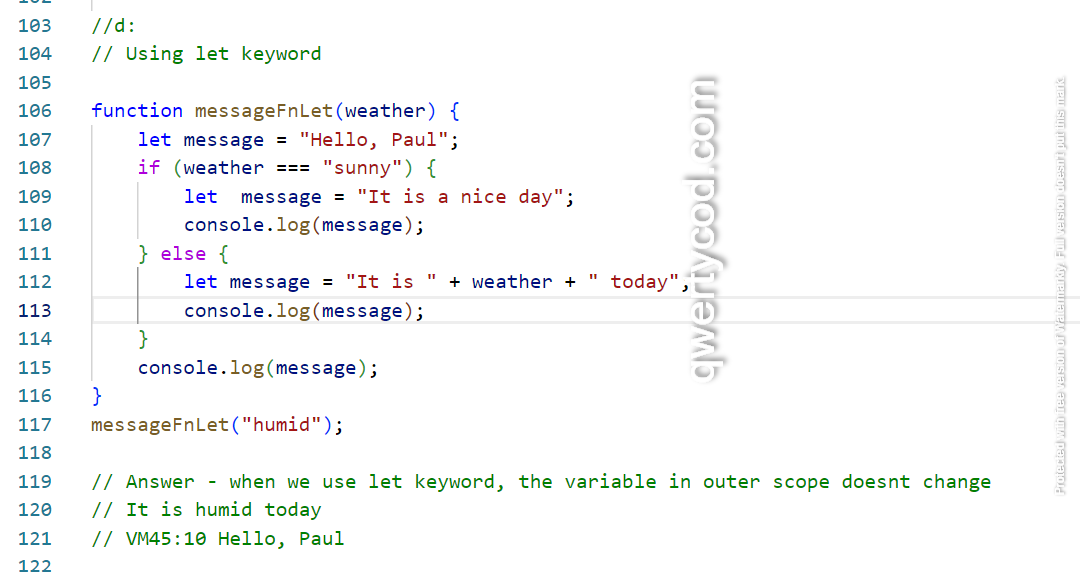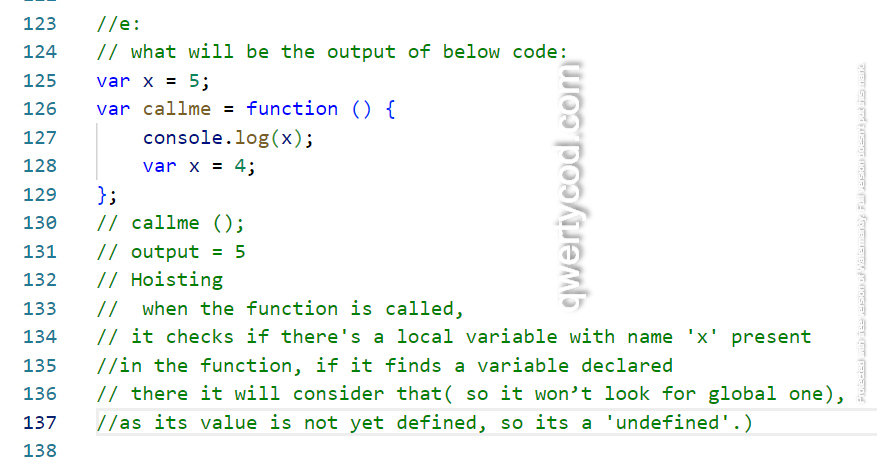5 => Use of Map method

Get employee name in capital from the input array.

let employees = [
{ Name: Alex Anderson, Salary: 50000, Age: 46 },
{ Name: Bob Brown, Salary: 5000, Age: 16 },
{ Name: Charles Smith, Salary: 35000, Age: 26 },
{ Name: Dreck Jones, Salary: 100000, Age: 36 }

];

Output should be –

[“ALEX ANDERSON”, “BOB BROWN”, “CHARLES SMITH”, “DRECK JONES”]

var output = employees.map(x => x.Name.toUpperCase())

6 => Get max salary of the employees

employees = [
{ Name: Alex Anderson, Salary: 50000, Age: 46 },
{ Name: Bob Brown, Salary: 5000, Age: 16 },
{ Name: Charles Smith, Salary: 35000, Age: 26 },
{ Name: Dreck Jones, Salary: 100000, Age: 36 }
];
var max = 0

employees.forEach(x=> { if(x => x.Salary > max){ max = x.Salary}});

7 => Get all substring from the string x
x = ‘abcd’
output = [‘a’, ‘ab’, ‘abc’, ‘abcd’, ‘b’, ‘bc’, ‘bcd’, ‘c’, ‘cd’, ‘d’]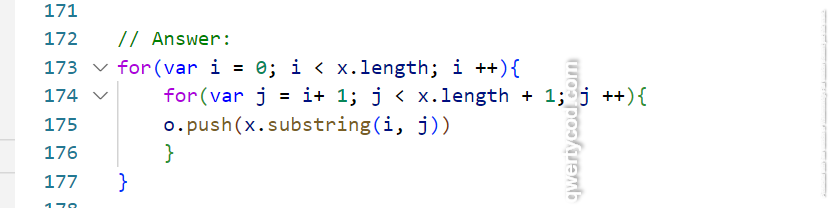8 => Shift left each character.
// input –  [ ‘a’,’b’, ‘c’, ‘d’, ‘e’]
// output should be –  [‘b’, ‘c’, ‘d’, ‘e’, ‘a’]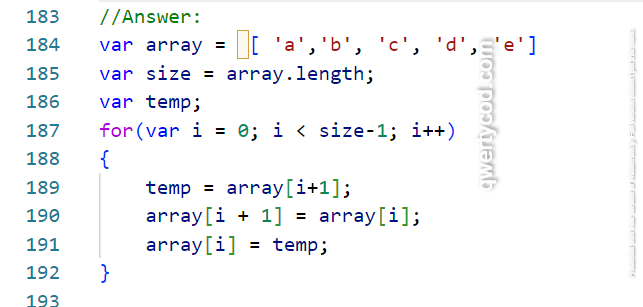9 => Degree between  hour and minute hands at any time.

Input for 8:45am
hour hand at 8, minutes hand at 45
Solution:
// In one hour, hour hand moves 180/12 = 30 degree
// In one minute, hour hand moves 30/60 = 0.5
// In one minute, minute hand moves 30/5 = 6 degree
// so at 3:15 pm – degress = (15*0.5) + (3*30) – (6*15) = 7.5
var degree = (minute*0.5) + (hour  * 30) – (6*minute)
10 => Find largest match from a array of word.
input array – [‘boo’, ‘ook’, ‘book’, ‘keyboard’, ‘mouse’, ‘ebook’,’computer’]
testing word – ‘comebooks’
output should be ‘ebook’